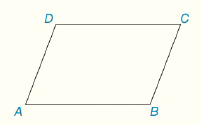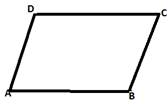Chapter 4.CT, Problem 1CT### Elementary Geometry for College St...

6th Edition
Daniel C. Alexander + 1 other
ISBN: 9781285195698

#### Solutions

Chapter
Section### Elementary Geometry for College St...

6th Edition
Daniel C. Alexander + 1 other
ISBN: 9781285195698
Textbook Problem
15 views

# Consider ▱ ABCD as shown.a) How are ∠ A and ∠ C related?b) How are ∠ A and ∠ B related?To determine

To Find:

The relation between A and C.

Solution:

A and C are congruent angles.

Explanation

Given parallelogram,From the property opposite angles in a parallelogram are congruent.

Since ABCD is a parallelogram, A and C are opposite angles then A and C are congruent angles.

The Final statement:

A and C are congruent angles.

To determine

To Find:

The relation between A and B.

Solution:

A and B are supplementary angles.

Explanation

Given parallelogram,From the property consecutive angles in a parallelogram are supplementary.

Since ABCD is a parallelogram, A and B are consecutive angles then A and B are supplementary angles.

The Final statement:

A and B are supplementary angles.

#### The Solution to Your Study Problems

Bartleby provides explanations to thousands of textbook problems written by our experts, many with advanced degrees!

Get Started

#### Find more solutions based on key concepts